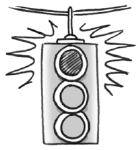### Home > GC > Chapter 10 > Lesson 10.2.2 > Problem10-73

10-73.Mrs. Cassidy solved the problem $\left(w-3\right)\left(w+5\right)=9$ and got $w = 3$ and $w=-5$. Is she correct? If so, show how you know. If not, show how you know and find the correct solution.

Substitute the solutions into the equation. Are the answers correct?

Rewrite the equation.

$w^2+2w-15=9$
$w^2+2w-24=0$

Factor the equation.

$\left(w+6\right)\left(w-4\right)=0$

$w=-6,4$